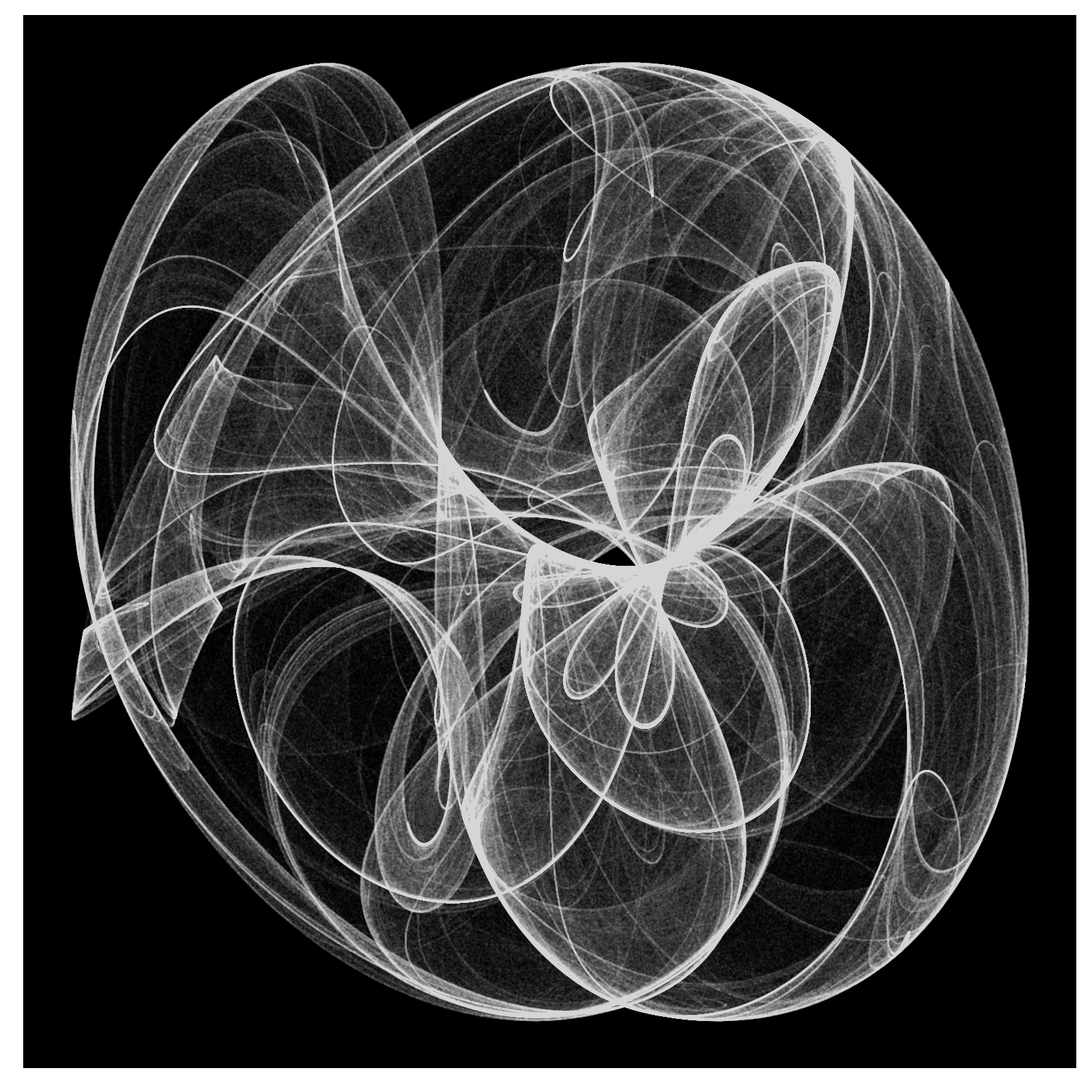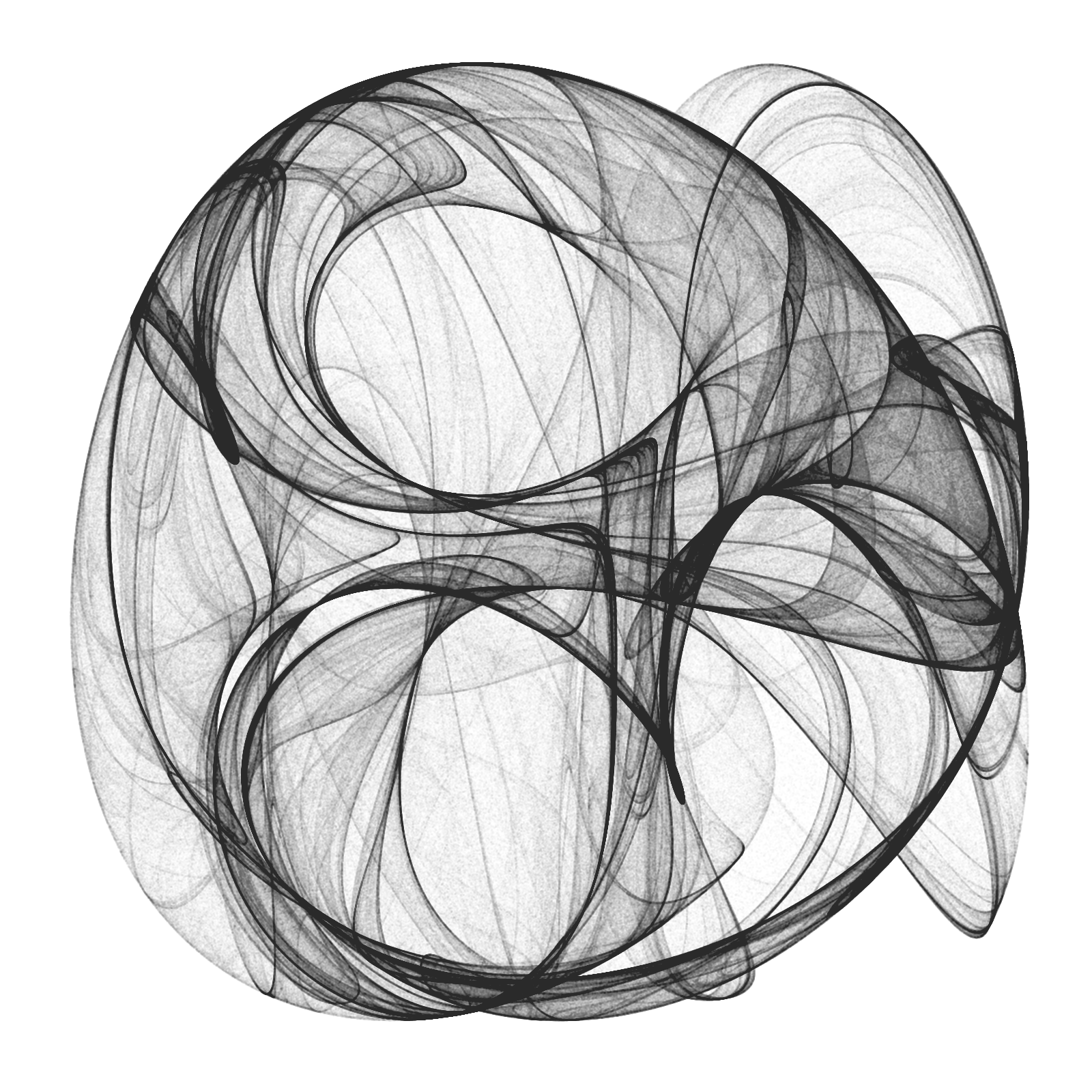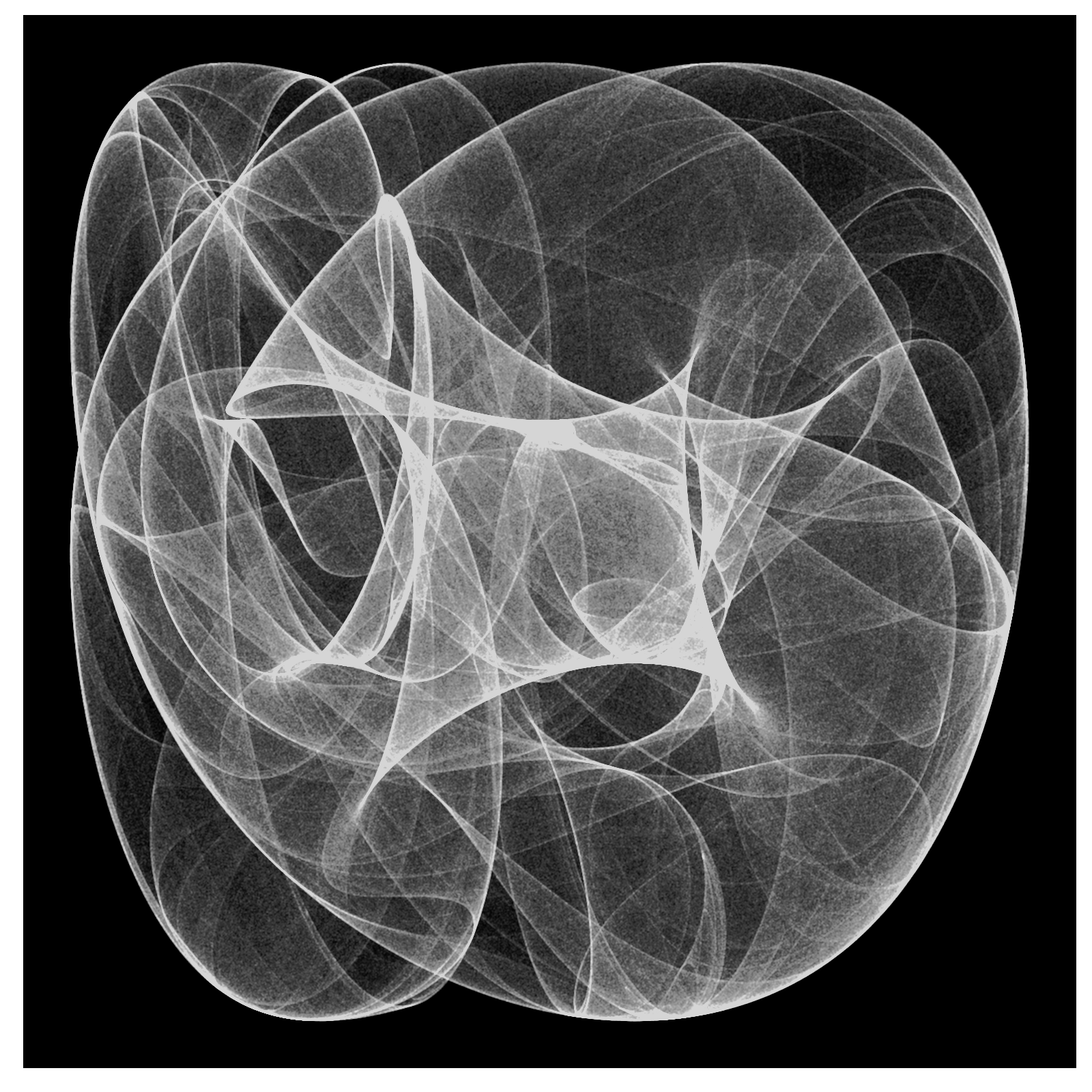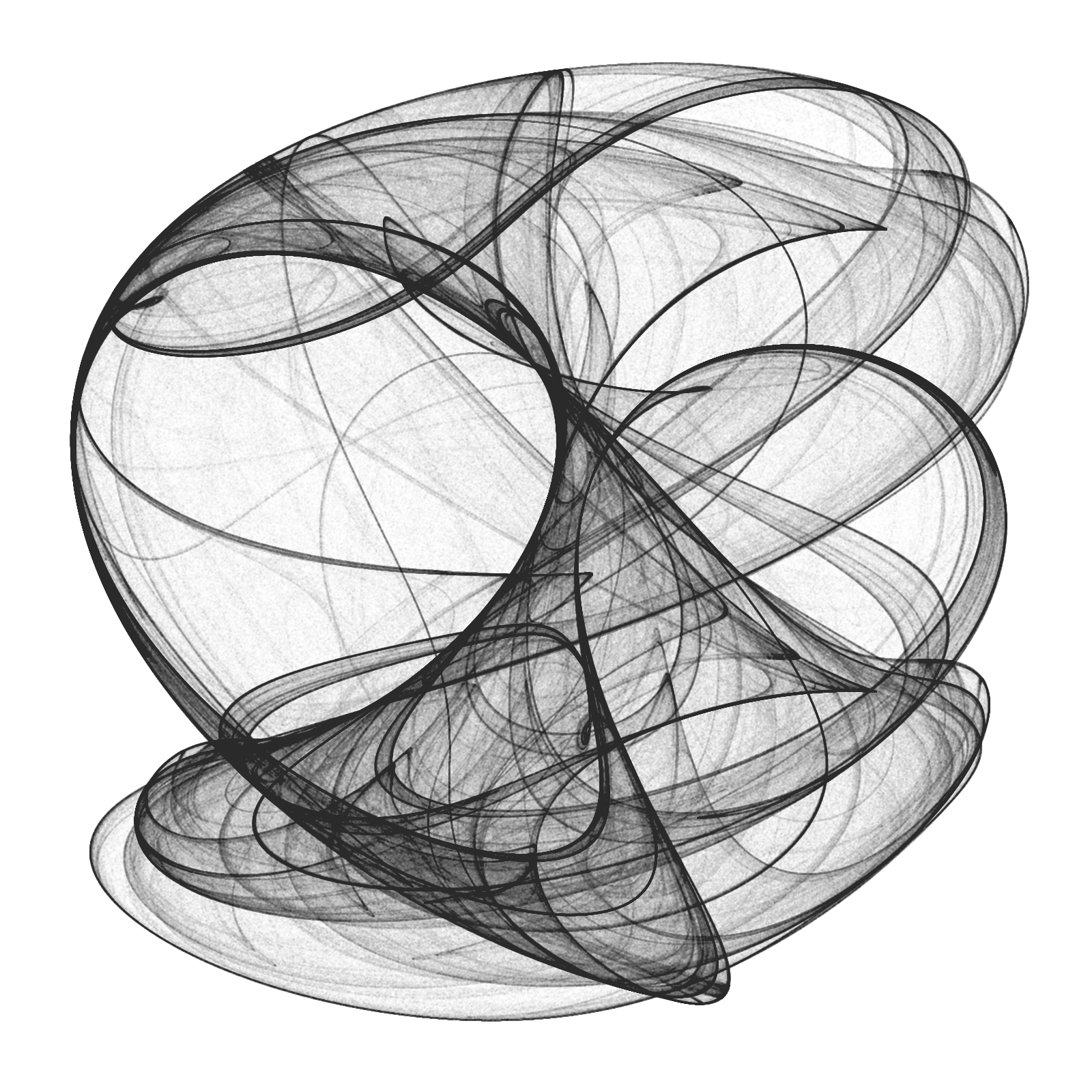# Abstractions

Spinning on that dizzy edge (Just Like Heaven, The Cure)

This post talks about a generative system called Physarum model, which simulates the evolution of a colony of extremely simple organisms that, under certain environmental conditions, result into complex behaviors. Apart from the scientific interest of the topic, this model produce impressive images like this one, that I call The Death of a Red Dwarf:

You can find a clear explanation of how a physarum model works in this post, by Sage Jenson. A much deeper explanation can be found in this paper by Jeff Jones, from the University of the West of England. Briefly, a physarum model evolves a set of particles (agents), making them move over a surface. Agents turn towards locations with higher concentrations of a pheromone trail. Once they move, they make a deposition of pheromone as well. These are the steps of a single iteration of the model:

• Sensor stage: Each agent looks to three positions of the trail map (left, front and right) according a certain sensor angle
• Motor stage: then it moves to the place with the higher concentration with some rules to deal with ties
• Deposition stage: once in the new location, the agent deposites a certain amount of pheromone.
• Diffuse stage: the pheromones diffusing over the surface to blur the trail array.
• Decay stage: this make to decay the concentration of pheromone on the surface.

Sage Jenson explains the process with this illustrative diagram:

My implementation is a bit different from Jones’ one. The main difference is that I do not apply the diffuse stage after deposition: I prefer a high defined picture instead blurriyng it. I also play with the initial arrangement of agents (location and heading angle) as well with the initial configuration of environment. For example, In The Death of a Red Dwarf, agents start from a circle and the environment is initialized in a dense disc. You can find the details in the code. There you will see that the system is governed by the following parameters:

• Front and left sensor angles from forward position
• Agent rotation angle
• Sensor offset distance
• Step size (how far agent moves per step)
• Chemoattractant deposition per step
• Trail-map chemoattractant diffusion decay factor

In adition to them (specific of the physarum model), you also can change others like colors, noise of angles (parameter amount of jitter function), number of agents and iterations as well as the initial arrangement of the environment and the location of agents. I invite you to do it. You will discover many abstractions: butterfly wings, planets, nets, explosions, supernovas … I have spent may hours playing with it. Some examples:

You can find the code here. Please, let me know if you do something interesting with it. Share your artworks with me in Twitter or drop me an email (you can find my address here).

# Watercolors

Do Sol de Ipanema
É mais que um poema
(Garota de Ipanema, João Gilberto)

Sometimes I think about the reasons why I spend so many time doing experiments and writing my discoveries in a blog. Even although the main reason to start this blog was some kind of vanity, today I have pretty clear why I still keep writing it: to keep my mind tuned. I really enjoy looking for ideas, learning new algorithms, figuring out the way to translate them into code and trying to discover new territories going a step further. I cannot imagine my life without coding. Many good times in the last years have been in front of my laptop listening music and drinking a beer. In these strange times, confined at house, coding has became in something more important. It keeps me ahead from the sad news and moves my mind to places where everything is quiet, friendly and perfect. Blogging is my therapy, my mindfulness.

This post is inspired in this post from Softology, an amazing blog I recommend you to read. In it, you can find a description of the stepping stone cellular automaton as well as a appealing collection of images generated using this technique. I modified the original algorithm described in the post to create images like these, which remind me a watercolor painting:

I begin with a 400 x 400 null matrix. After that, I choose a number of random pixels that will act as centers of circles. Around them I substitute the initial zeros by numbers drawned from a normal distribution which mean depends on the distance of pixels to the center. The next step is to apply the stepping stone algorithm. For each pixel, I substitute its value by a weighted average of itself and the value of some of its neighbors, choosen randomly. I always mix values of the pixels. The original algorithm, as described in the Softology’s blog, performs these mixings randomly. Another difference is that I mix values intead interchanging them, as the original algorithm does. Once I repeat this process a number of times, I pick a nice palette from COLOURLovers and turn values of pixels into colors with ggplot:

The code is here. Let me know if you do something interesting with it. Turning numbers into bright colors: I cannot imagine a better way to spend some hours in these shadowy times.

# Reaction Diffusion

Sin patria ni banderas, ahora vivo a mi manera; y es que me siento extranjero fuera de tus agujeros (Tercer movimiento: Lo de dentro, Extremoduro)

The technique I experimented with in this post is an endless source to obtain amazing images. It is called reaction-diffusion and simulates the evolution of a system where several substances interact chemically transforming into each other (reaction) and spreading out over a surface in space (diffusion). In my case there are just two substances in a 2D space and the evolution of system is simulated using the Gray-Scott algorithm which is ruled by several parameters that, once determined, can produce images like this one:

This article by Karl Sims, is a very clear and comprehensive explanation of the Gray-Scott algorithm. Briefly, the Gray-Scott model simulates the evolution of two substances, A and B in a two dimensional grid. In each cell of the grid, the substance A is added at a given feed rate f. Then, both substances react following this rule: two particles of B convert a particle of A into a particle of B. To prevent overpopulation, B particles are killed at a given kill rate k. In the diffusion phase, both substances spread out accross the cells of the grid at a given diffusion rates Da and Db. The diffusion is performed using a 2D Lapacian operator with a 3x3 convolution matrix L.

In the article you can find the equations that rule the system, which depend on the parameters described in the previous paragraph. To obtain all the images of this post, I maintained most of them always constant and just changed the following:

• Feed and kill rates (f and k respectively)
• The initial proportion of both substances A and B. I always started with A=0 in each cell and B=1 in some (small) amount of them selected randomly or according to some geometrical considerations (and inner circle or square, for example). I let you to try with your own ideas.

Sometimes the system converges and remains stable after a number of iterations. For example, these images are obtained iterating 5000 times:

Before converging you can obtain also nice patterns in between:

The variety of patterns is amazing: tessellations, lattices, caleidoscopic … some more examples:

I used again Rcpp to iterate efficiently but now I tried RcppArmadillo, a C++ library for linear algebra and scientific computing because it contains a class called cube, which is a 3D matrix that fits perfectly into a 2D grid where the 3rd dimension is the amount of particles A and B.

I like to share my code because I think it may serve as a source of inspiration to someone. You can find the code here with just one of the many configurations I tried to generate the previous images. It may serve you as a good starting point to explore you own ones. Let me know if you reach interesting places.

Happy New Year 2020.

# The Chaos Game: an experiment about fractals, recursivity and creative coding

Mathematics, rightly viewed, possesses not only truth, but supreme beauty (Bertrand Russell)

You have a pentagon defined by its five vertex. Now, follow these steps:

• Step 0: take a point inside the pentagon (it can be its center if you want to do it easy). Keep this point in a safe place.
• Step 1: choose a vertex randomly and take the midpoint between both of them (the vertex and the original point). Keep also this new point. Repeat Step 1 one more time.
• Step 2: compare the last two vertex that you have chosen. If they are the same, choose another with this condition: if it’s not a neighbor of the last vertex you chose, keep it. If it is a neighbor, choose another vertex randomly until you choose a not-neighbor one. Then, take the midpoint between the last point you obtained and this new vertex. Keep also this new point.
• Step 3: Repeat Step 2 a number of times and after that, do a plot with the set of points that you obtained.

If you repeat these steps 10 milion times, you will obtain this stunning image:

I love the incredible ability of maths to create beauty. More concretely, I love the fact of how repeating extremely simple operations can bring you to unexpected places. Would you expect that the image created with the initial naive algorithm would be that? I wouldn’t. Even knowing the result I cannot imagine how those simple steps can produce it.

The image generated by all the points repeat itself at different scales. This characteristic, called self-similarity, is property of fractals and make them extremely attractive. Step 2 is the key one to define the shape of the image. Apart of comparing two previous vertex as it’s defined in the algorithm above, I implemented two other versions:

• one version where the currently chosen vertex cannot be the same as the previously chosen vertex.
• another one where the currently chosen vertex cannot neighbor the previously chosen vertex if the three previously chosen vertices are the same (note that this implementation is the same as the original but comparing with three previous vertex instead two).

These images are the result of applying the three versions of the algorithm to a square, a pentagon, a hexagon and a heptagon (a row for each polygon and a column for each algorithm):

From a technical point of view I used Rcppto generate the set of points. Since each iteration depends on the previous one, the loop cannot easily vectorised and C++ is a perfect option to avoid the bottleneck if you use another technique to iterate. In this case, instead of writing the C++ directly inside the R file with cppFunction(), I used a stand-alone C++ file called chaos_funcs.cpp to write the C++ code that I load into R using sourceCpp().

Some days ago, I gave a tutorial at the coding club of the University Carlos III in Madrid where we worked with the integration of C++ and R to create beautiful images of strange attractors. The tutorial and the code we developed is here. You can also find the code of this experiment here. Enjoy!

# Mandalaxies

One cannot escape the feeling that these mathematical formulas have an independent existence and an intelligence of their own, that they are wiser than we are, wiser even than their discoverers (Heinrich Hertz)

I love spending my time doing mathematics: transforming formulas into drawings, experimenting with paradoxes, learning new techniques … and R is a perfect tool for doing it. Maths are for me a the best way of escape and evasion from reality. At least, doing maths is a stylish way of wasting my time.

When I read something interesting, many times I feel the desire to try it by myself. That’s what happened to me when I discovered this fabolous book by Julien C. Sprott. I cannot stop doing images with the formulas that contains. Today I present you a mix of mandalas and galaxies that I called Mandalaxies:

This time, the equation that drives these drawings is this one:$x_{n+1}= 10a_1+(x_n+a_2sin(a_3y_n+a_4))cos(\alpha)+y_nsin(\alpha)\\ y_{n+1}= 10a_5-(x_n+a_2sin(a_3y_n+a_4))sin(\alpha)+y_nsin(\alpha)$
where$\alpha=2\pi/(13+10a_6)$

The equation depends on six parameters (from a1 to a6). Searching randomly for values between -1.2 and 1.3 to each of them, you can generate an infinite number of beautiful images:

Here you can find the code to do your own images. Once again, Rcpp is key to generate the set of points to plot quickly since each of the previous plots contains 4 million points.

# Rcpp, Camarón de la Isla and the Beauty of Maths

Desde que te estoy queriendo
yo no sé lo que me pasa
cualquier vereda que tomo
siempre me lleva a tu casa
(Y mira que mira y mira, Camarón de la Isla)

The verses that head this post are taken from a song of Camarón de la Isla and illustrate very well what is a strange attractor in the real life. For non-Spanish speakers a translation is since I’m loving you, I don’t know what happens to me: any path I take, always ends at your house. If you don’t know who is Camarón de la Isla, hear his immense and immortal music.

I will not try to give here a formal definition of a strange attractor. Instead of doing it, I will try to describe them with my own words. A strange attractor can be defined with a system of equations (I don’t know if all strage attractors can be defined like this). These equations determine the trajectory of some initial point along a number of steps. The location of the point at step i, depends on the location of it at step i-1 so the trajectory is calculated sequentially. These are the equations that define the attractor of this experiment:$x_{n+1}= a_{1}+a_{2}x_{n}+a_{3}y_{n}+a_{4} |x_{n}|^{a_5}+a_{6} |y_{n}|^{a_7}\\ y_{n+1}= a_{8}+a_{9}x_{n}+a_{10}y_{n}+a_{11} |x_{n}|^{a_{12}}+a_{13} |y_{n}|^{a_{14}}$

As you can see there are two equations, describing the location of each coordinate of the point (therefore it is located in a two dimensional space). These equations are impossible to resolve. In other words, you cannot know where will be the point after some iterations directly from its initial location. The adjective attractor comes from the fact of the trajectory of the point tends to be the same independently of its initial location.

Here you have more examples: folds, waterfalls, sand, smoke … images are really appealing:

The code of this experiment is here. You will find there a definition of parameters that produce a nice example image. Some comments:

• Each point depends on the previous one, so iteration is mandatory; since each plot involves 10 million points, a very good option to do it efficiently is to use Rcpp, which allows you to iterate directly in C++.
• Some points are quite isolated and far from the crowd of points. This is why I locate some breakpoints with quantile to remove tails. If not, the plot may be reduced to a big point.
• The key to obtain a nice plot if to find out a good set of parameters (a1 to a14). I have my own method, wich involves the following steps: generate a random value for each between -4 and 4, simulate a mini attractor of only 2000 points and keep it if it doesn’t diverge (i.e. points don’t go to infinite), if x and y are not correlated at all and its kurtosis is bigger than a certain thresold. If the mini attractor overcome these filters, I keep its parameters and generate the big version with 10 million points.
• I would have publish this method together with the code but I didn’t. Why? Because this may bring yourself to develop your own since mine one is not ideal. If you are interested in mine, let me know and I will give you more details. If you develop a good method by yourself and don’t mind to share it with me, let me know as well, please.

This post is inspired in this beautiful book from Julien Clinton Sprott. I would love to see your images.

# Drawing 10 Million Points With ggplot: Clifford Attractors

For me, mathematics cultivates a perpetual state of wonder about the nature of mind, the limits of thoughts, and our place in this vast cosmos (Clifford A. Pickover – The Math Book: From Pythagoras to the 57th Dimension, 250 Milestones in the History of Mathematics)

I am a big fan of Clifford Pickover and I find inspiration in his books very often. Thanks to him, I discovered the harmonograph and the Parrondo’s paradox, among many other mathematical treasures. Apart of being a great teacher, he also invented a family of strange attractors wearing his name. Clifford attractors are defined by these equations:$x_{n+1}\, =\, sin(a\, y_{n})\, +\, c\, cos(a\, x_{n}) \\ y_{n+1}\, =\, sin(b\, x_{n})\, +\, d\, cos(b\, y_{n}) \\$

There are infinite attractors, since a, b, c and d are parameters. Given four values (one for each parameter) and a starting point (x0, y0), the previous equation defines the exact location of the point at step n, which is defined just by its location at n-1; an attractor can be thought as the trajectory described by a particle. This plot shows the evolution of a particle starting at (x0, y0)=(0, 0) with parameters a=-1.24458046630025, b=-1.25191834103316, c=-1.81590817030519 and d=-1.90866735205054 along 10 million of steps:Changing parameters is really entertaining. Drawings have a sandy appearance:From a technical point of view, the challenge is creating a data frame with all locations, since it must have 10 milion rows and must be populated sequentially. A very fast way to do it is using Rcpp package. To render the plot I use ggplot, which works quite well. Here you have the code to play with Clifford Attractors if you want:

library(Rcpp)
library(ggplot2)
library(dplyr)

opt = theme(legend.position  = "none",
panel.background = element_rect(fill="white"),
axis.ticks       = element_blank(),
panel.grid       = element_blank(),
axis.title       = element_blank(),
axis.text        = element_blank())

cppFunction('DataFrame createTrajectory(int n, double x0, double y0,
double a, double b, double c, double d) {
// create the columns
NumericVector x(n);
NumericVector y(n);
x=x0;
y=y0;
for(int i = 1; i < n; ++i) {
x[i] = sin(a*y[i-1])+c*cos(a*x[i-1]);
y[i] = sin(b*x[i-1])+d*cos(b*y[i-1]);
}
// return a new data frame
return DataFrame::create(_["x"]= x, _["y"]= y);
}
')

a=-1.24458046630025
b=-1.25191834103316
c=-1.81590817030519
d=-1.90866735205054

df=createTrajectory(10000000, 0, 0, a, b, c, d)

png("Clifford.png", units="px", width=1600, height=1600, res=300)
ggplot(df, aes(x, y)) + geom_point(color="black", shape=46, alpha=.01) + opt
dev.off()